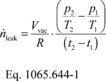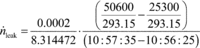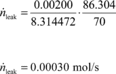# Title 40

## SECTION 1065.644

### 1065.644 Vacuum-decay leak rate.

§ 1065.644 Vacuum-decay leak rate.

This section describes how to calculate the leak rate of a vacuum-decay leak verification, which is described in § 1065.345(e). Use the following equation to calculate the leak rate n leak, and compare it to the criterion specified in § 1065.345(e):Where: Vvac = geometric volume of the vacuum-side of the sampling system. R = molar gas constant. p2 = vacuum-side absolute pressure at time t2. T2 = vacuum-side absolute temperature at time t2. p1 = vacuum-side absolute pressure at time t1. T1 = vacuum-side absolute temperature at time t1. t2 = time at completion of vacuum-decay leak verification test. t1 = time at start of vacuum-decay leak verification test. Example: Vvac = 2.0000 L = 0.00200 m 3 R = 8.314472 J/(mol · K) = 8.314472 (m 2 · kg)/(s 2 · mol · K) p2 = 50.600 kPa = 50600 Pa = 50600 kg/(m · s 2) T2 = 293.15 K p1 = 25.300 kPa = 25300 Pa = 25300 kg/(m · s 2) T1 = 293.15 K t2 = 10:57:35 a.m. t1 = 10:56:25 a.m.[79 FR 23795, Apr. 28, 2014]